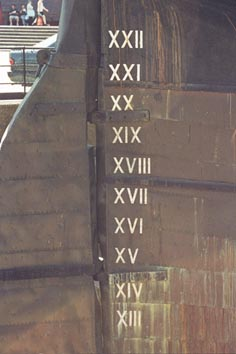# Roman Numerals

## Roman Numerals are the symbols that were used in Ancient Rome for counting and are a combination of letters from the Latin alphabet - I, V, X, L, C, D and M.

The Ancient Romans had no concept of zero or of place value. They assigned values to the seven letters and these were arranged in a specific order to represent numbers.

# I = 1 V = 5 X = 10 L = 50 C = 100 D = 500 M = 1000

Roman numerals are added when grouped together,

II = 2 (1 + 1), XX = 20 (10 + 10) etc

## No more than three identical letters can be placed together.

So, I = 1, II = 2, III = 3

How do we make 4? IIII would have too many identical letters

## If a smaller value appears to the left of a larger value, the smaller value is subtracted from the larger.

So IV = 4 (V-I or 5 - 1), IX = 9 (X-I or 10 - 1)

## If a smaller value appears to the right of a larger value, the smaller value is added to the larger.

So VI = 6 (V+I or 5 + 1), VII = 7 (V+I+I or 5 + 1 + 1), XI = 11 (X+I or 10 + 1)

## How to convert decimal numbers to Roman Numerals

First use your knowledge of place value to partition the number into thousands, hundreds, tens and units, so 1987 would be 1000 + 900 + 80 + 7

Next convert these values into Roman Numerals,

1000 is M, 900 is CM (1000-100), 80 is LXXX (50 + 10 + 10 + 10) and 7 is VII (5 + 1 + 1)

Put these letters together - MCMLXXXVII

## How to convert Roman Numerals to decimal numbers

First we have to work out the groupings, remembering the rules, especially that if a smaller value is to the left of a larger value then the smaller value is subtracted from the larger.

Lets take MDCCCLVI, that is M DCCC L VI

M is 1000, DCCC is 800 (500 + 100 + 100 + 100), L is 50 and VI is 6 (5 + 1)

1000 + 800 + 50 + 6 = 1856Big Ben clockfaceStern of the Cutty Sark

We still use Roman Numerals today, for:

The date on films and TV programmes

To show the hours on clocks (Big Ben) and watches

For sporting events like the Olympics
(The Games of the MMXVI Olympiad)

For Kings and Queens (King Henry VIII) and Popes.

Have your child look out for examples of the use of Roman Numerals and get them to convert them.

Work out their and the rest of the families birthday years in Roman Numerals

To check these conversions, you could use this converter

Roman Numerals Converter